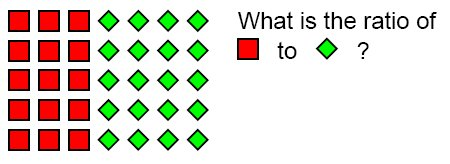Model Ratios
Multiplication Tables
Are the ratios equivalent?
Solve Equivalent Ratios
100

Write a Ratio 3 ways of yellow to red circles:3/4, 3:4, 3 to 4

100

Write 3 more Equivalent Ratios using a multiplication table:

1/4

2/8, 3/12, 4/16

100

Are the Ratios Equivalent?

Show Evidence and write Yes or No.

2/4 = 3/5

10 = 12

No!

100

Solve for x to make the Ratios Equivalent:

x/5 = 8/10

x = 4

200

Write a ratio 3 ways of yellow to red circles:5/3, 5:3, 5 to 3

200

Write 3 more Equivalent Ratios using a multiplication table:

2/9

4/18, 6/27, 8/36

200

Are the Ratios Equivalent?

Show Evidence and write Yes or No.

5/10 = 3/6

30 = 30

Yes!

200

Solve for x to make the Ratios Equivalent:

3/9 = 9/x

x = 27

300

Write a ratio 3 ways of red to blue circles:5/6, 5:6, 5 to 6

300

Write 3 more Equivalent Ratios using a multiplication table:

4/7

8/14, 12/21, 16/28

300

Are the Ratios Equivalent?

Show Evidence and write Yes or No.

8/10 = 6/8

64 = 60

No!

300

Solve for x to make the Ratios Equivalent:

7/4 = x/12

x = 21

400

Write a ratio 3 ways of squares to diamonds:15:20, 15:20, 15 to 20

400

Write 3 more Equivalent Ratios using a multiplication table:

3/8

6/16, 9/24, 12/32

400

Are the Ratios Equivalent?

Show Evidence and write Yes or No.

3/4 = 9/12

36 = 36

Yes!

400

Solve for x to make the Ratios Equivalent:

2/6 = x/30

x = 10

500

Write a ratio 3 ways of Circles to Squares:10/4, 10:4, 10 to 4

500

Write 3 more Equivalent Ratios using a multiplication table:

11/12

22/24, 33/36, 44/48

500

Are the Ratios Equivalent?

Show Evidence and write Yes or No.

8/3 = 32/12

96 = 96

Yes!

500

Solve for x to make the Ratios Equivalent:

10/x = 4/12

x = 30

Click to zoom